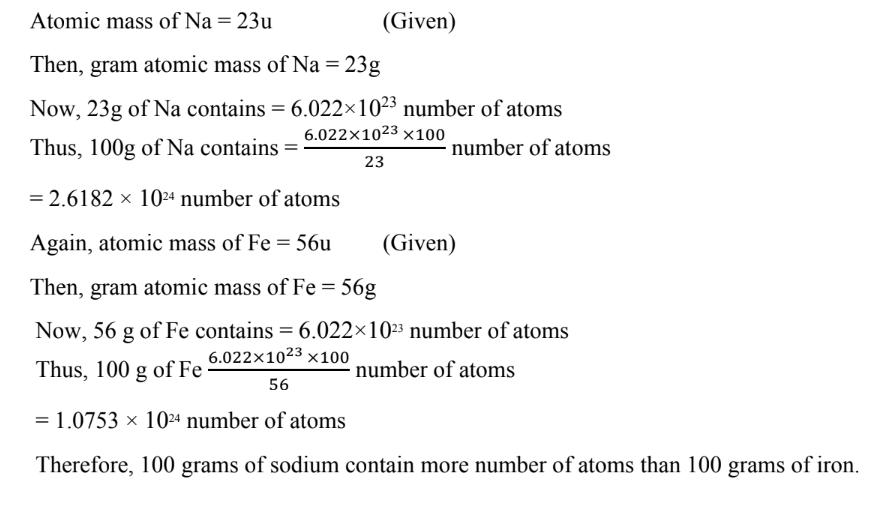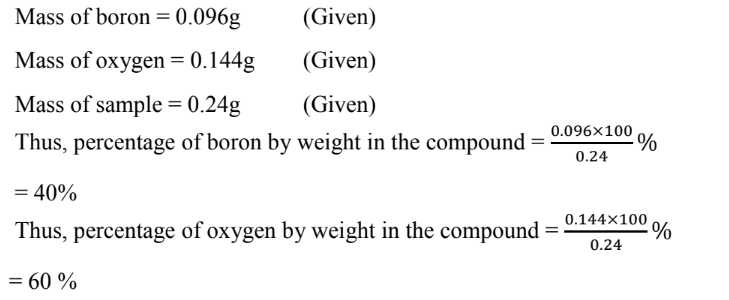Class IX Science

# Chapter - 3 Atoms and Molecules

## NCERT Solutions for Class 9 Science

Other Chapters
In page question - Page 32
Q 1.

In a reaction 5.3 g of sodium carbonate reacted with 6 g of ethanoic acid. The products were 2.2 g

of carbon dioxide, 0.9g water and 8.2 g of sodium ethanoate. Show that these observations are in agreement with the law of conservation of mass.

Sodium carbonate + ethanoic acid → sodium ethanoate + carbon dioxide + water

Ans.

In a reaction, sodium carbonate reacts with ethanoic acid to produce sodium ethanoate, carbondioxide, and water.

Sodium + Ethanoic → Sodium + Carbon + Water

Carbonate acid ethanoate dioxide

Mass of sodium carbonate = 5.3g      (Given)

Mass of ethanoic acid = 6g                (Given)

Mass of sodium ethanoate = 8.2g     (Given)

Mass of carbon dioxide = 2.2            (Given)

Mass of water = 0.9g                         (Given)

Now, total mass before the reaction = (5.3 + 6)g = 11. 3g

and total mass after the reaction = (8.2 + 2.2 + 0.9)g =  11.3g

Therefore, Total mass before the reaction = Total mass after the reaction

Hence, the given observations are in agreement with the law of conservation of mass.

In page question - Page 33
Q 1.

Hydrogen and oxygen combine in the ratio of 1 : 8 by mass to form water. What mass of oxygen gas would be required to react completely with 3g of hydrogen gas?

Ans.

It is given that the ratio of hydrogen and oxygen by mass to form water is 1:8. Then, the mass of oxygen gas required to react completely with 1g of hydrogen gas is 8g. Therefore, the mass of oxygen gas required to react completely with 3g of hydrogen gas is 8 × 3g = 24 g.

In page question - Page 33
Q 2.

Which postulate of Dalton's atomic theory is the result of the law of conservation of mass?

Ans.

The postulate of Dalton's atomic theory which is a result of the law of conservation of mass is

“Atoms are indivisible particles, which can neither be created nor destroyed in a chemical

reaction”.

In page question - Page 33
Q 3.

Which postulate of Dalton's atomic theory can explain the law of definite proportions?

Ans.

The postulate of Dalton's atomic theory which can explain the law of definite proportion is “The relative number and kind of atoms in a given compound remains constant”.

In page question - Page 35
Q 1.

Define atomic mass unit.

Ans.

Mass unit equal to exactly one- twelfth the mass of one atom of carbon - 12 is called one atomic mass unit. It is written as 'u'.

In page question - Page 35
Q 2.

Why is it not possible to see an atom with naked eyes?

Ans.

The size of an atom is so small that it is not possible to see it with naked eyes. Also, atom of an element does not exist independently.

In page question - Page 39
Q 1.

Write down the formula of

(i) sodium oxide

(ii) aluminium chloride

(iii) sodium suphide

(iv) magnesium hydroxide

Ans.

(i) Sodium oxide → Na2O

(ii) Aluminium chloride → AlCl3

(iii) Sodium suphide → Na2S

(iv) Magnesium hydroxide → Mg(OH)2

In page question - Page 39
Q 2.

Write down the names of compounds represented by the following formula:

(i) Al2(SO4)3

(ii) CaCl2

(iii) K2SO4

(iv) KNO3

(v) CaCO3

Ans.

(i) Al(SO4)3→ Aluminium sulphate

(ii) CaCl2→ Calcium chloride

(iii) K2SO4→ Potassium sulphate

(iv) KNO→ Potassium Nitrate

(v) CaCO3→ Calcium carbonate

In page question - Page 39
Q 3.

What is meant by the term chemical formula?

Ans.

The chemical formula of a compound means the symbolic representation of the composition of a compound. From the chemical formula of a compound, we can know the number and kinds of atoms of different elements that constitute the compound. For example, from the chemical formula CO2 of carbon dioxide, we come to know that one carbon atom and two oxygen atoms are chemically bonded together to form one molecule of the compound, carbon dioxide.

In page question - Page 39
Q 4.

How many atoms are present in a

(i) H2S molecule and

(ii) PO43-  ion?

Ans.

(i) In an H2S molecule, three atoms are present; two of hydrogen and one of sulphur.

(ii) In a PO43- ion, five atoms are present; one of phosphorus and four of oxygen.

In page question - Page 40
Q 1.

Calculate the molecular masses of H2, O2, Cl2, CO2, CH4, C2H6, C2H4, NH3, CH3OH.

Ans.

Molecular mass of H2 = 2 × Atomic mass of H

= 2 × 1 = 2u

Molecular mass of O2 = 2 × Atomic mass of O

= 2 × 16 = 32u

Molecular mass of Cl2 = 2 × Atomic mass of Cl

= 2 × 35.5 = 71 u

Molecular mass of CO2 = Atomic mass of C + 2 × Atomic mass of O

= 12 + 2 × 16 = 44 u

Molecular mass of CH4 = Atomic mass of C + 4 × Atomic mass of H

= 12 + 4 × 1 = 16 u

Molecular mass of C2H6 = 2 × Atomic mass of C + 6 × Atomic mass of H

= 2 × 12 + 6 × 1 = 30u

Molecular mass of C2H4 = 2 × Atomic mass of C + 4 × Atomic mass of H

= 2 × 12 + 4 × 1 = 28u

Molecular mass of NH3 = Atomic mass of N + 3 × Atomic mass of H

= 14 + 3 × 1 =17 u

Molecular mass of CH3OH Atomic mass of C+4 ×Atomic mass of H+Atomic mass of O

= 12 + 4 × 1 + 16 = 32 u

In page question - Page 40
Q 2.

Calculate the formula unit masses of ZnO, Na2O, K2CO3, given masses of Zn = 65u, Na = 23u, K

= 39u, C = 12u, and O = 16u.

Ans.

Formula unit mass of ZnO = Atomic mass of Zn + Atomic mass of O

= 65 + 16 = 81 u

Formula unit mass of Na2O = 2 × Atomic mass of Na + Atomic mass of O

= 2 × 23 + 16 = 62u

Formula unit mass of K2CO3

= 2 × Atomic mass of K + Atomic mass of C + 3 × Atomic mass of O

= 2 × 39 + 12 + 3 × 16 = 138u

In page question - Page 42
Q 1.

If one mole of carbon atoms weighs 12 gram, what is the mass (in gram) of 1 atom of carbon?

Ans.Chapter end exercise
Q 1.

A 0.24 g sample of compound of oxygen and boron was found by analysis to contain 0.096 g if boron and 0.144 g of oxygen. Calculate the percentage composition of the compound by weight.

Ans.Chapter end exercise
Q 2.

When 3.0 g of carbon is burnt in 8.00 g oxygen, 11.00 g of carbon dioxide is produced. What mass of carbon dioxide will be formed when 3.00 g of carbon is burnt in 50.00 g of oxygen? Which law of chemical combinations will govern your answer?

Ans.

Carbon + Oxygen → Carbon dioxide

3g of carbon reacts with 8 g of oxygen to produce 11g of carbon dioxide. If 3g of carbon is burnt in 50g of oxygen, then 3g of carbon will react with 8 g of oxygen. The remaining 42 g of oxygen will be left un-reactive. In this case also, only 11g of carbon dioxide will be formed. The above answer is governed by the law of constant proportions.

Chapter end exercise
Q 3.

What are polyatomic ions? Give examples?

Ans.

A polyatomic ion is a group of atoms carrying a charge (positive or negative).

For example, ammonium ion (NH4+), hydroxide ion (OH), carbonate ion (CO32−), sulphateion (SO42−).

Chapter end exercise
Q 4.

Write the chemical formula of the following:

(a) Magnesium chloride

(b) Calcium oxide

(c) Copper nitrate

(d) Aluminium chloride

(e) Calcium carbonate

Ans.

(a) Magnesium chloride →MgCl2

(b) Calcium oxide →CaO

(c) Copper nitrate →Cu(NO3)2

(d) Aluminium chloride →AlCl3

(e) Calcium carbonate →CaCO3

Chapter end exercise
Q 5.

Give the names of the elements present in the following compounds:

(a) Quick lime

(b) Hydrogen bromide

(c) Baking powder

(d) Potassium sulphate.

Ans.
 Compound Chemical formula Elements present Quick lime CaO Calcium, oxygen Hydrogen bromide HBr Hydrogen, bromine Baking powder NaHCO3 Sodium, hydrogen, carbon, oxygen Potassium sulphate K2SO4 Potassium, sulphur, oxygen
Chapter end exercise
Q 6.

Calculate the molar mass of the following substances:

(a) Ethyne, C2H2

(b) Sulphur molecule, S8

(c) Phosphorus molecule, P4 (atomic mass of phosphorus = 31)

(d) Hydrochloric acid, HCl

(e) Nitric acid, HNO3

Ans.

(a) Molar mass of ethyne, C2H2 = 2 × 12 + 2 × 1 = 28g

(b) Molar mass of sulphur molecule, S8 = 8 × 32 = 256g

(c) Molar mass of phosphorus molecule, P4 = 4 × 31 = 124g

(d) Molar mass of hydrochloric acid, HCl = 1 + 35.5 = 36.5g

(e) Molar mass of nitric acid, HNO3 = 1 + 14 + 3 × 16 = 63g

Chapter end exercise
Q 7.

What is the mass of

(a) 1 mole of nitrogen atoms?

(b) 4 mole of aluminium atoms (Atomic mass of aluminium = 27)?

(c) 10 moles of sodium sulphite (Na2SO3)?

Ans.

(a) The mass of 1 mole of nitrogen atoms is 14g.

(b) The mass of 4 moles of aluminium atoms is (4 × 27)g = 108g

(c) The mass of 10 moles of sodium sulphite (Na2SO3) is 10 × [2 × 23 + 32 + 3 × 16]g = 10 × 126g = 1260g

Chapter end exercise
Q 8.

Convert into mole.

(a) 12g of oxygen gas

(b) 12g of water

(c) 22g of carbon dioxide

Ans.

(a) 32 g of oxygen gas = 1 mole

Then, 12g of oxygen gas = 12/32 mole = 0.375 mole

(b) 18g of water = 1 mole

Then, 20 g of water = 20/18 mole = 1.11 moles (approx.)

(c) 44g of carbon dioxide = 1 mole

Then, 22g of carbon dioxide = 22/44 mole = 0.5 mole

Chapter end exercise
Q 9.

What is the mass of:

(a) 0.2 mole of oxygen atoms?

(b) 0.5 mole of water molecules?

Ans.

(a) Mass of one mole of oxygen atoms = 16g

Then, mass of 0.2 mole of oxygen atoms = 0.2 × 16g = 3.2g

(b) Mass of one mole of water molecule = 18g

Then, mass of 0.5 mole of water molecules = 0.5 × 18g = 9g

Chapter end exercise
Q 10.

Calculate the number of molecules of sulphur (S8) present in 16g of solid sulphur.

Ans.

1 mole of solid sulphur (S8) = 8 × 32g = 256g

i.e., 256g of solid sulphur contains = 6.022 × 1023 molecules

Then, 16g of solid sulpur contains  x  16 molecules

= 3.76 × 1022 molecules (approx)

Chapter end exercise
Q 11.

Calculate the number of aluminium ions present in 0.051g of aluminium oxide.

(Hint: The mass of an ion is the same as that of an atom of the same element. Atomic mass of Al = 27u)

Ans.

1 mole of aluminium oxide (Al2O3) = 2 × 27 + 3 × 16 = 102g

i.e., 102g of Al2O3 = 6.022 × 1023 molecules of Al2O3

Then, 0.051 g of Al2O3 contains =  × 0.051 molecules

= 3.011 × 1020 molecules of Al2O3

The number of aluminium ions (Al3+) present in one molecules of aluminium oxide is 2.

Therefore, The number of aluminium ions (Al3+) present in

3.11 × 1020 molecules (0.051g) of aluminium oxide (Al2O3) = 2 × 3.011 × 1020

= 6.022 × 1020